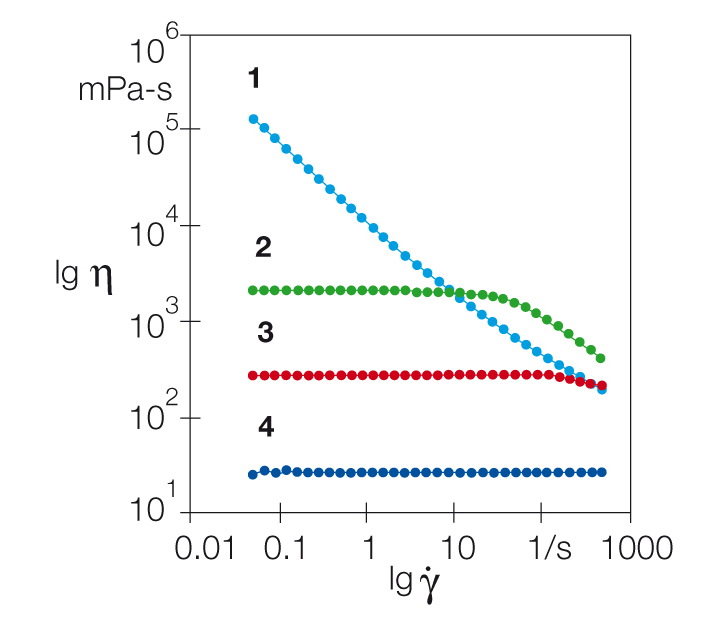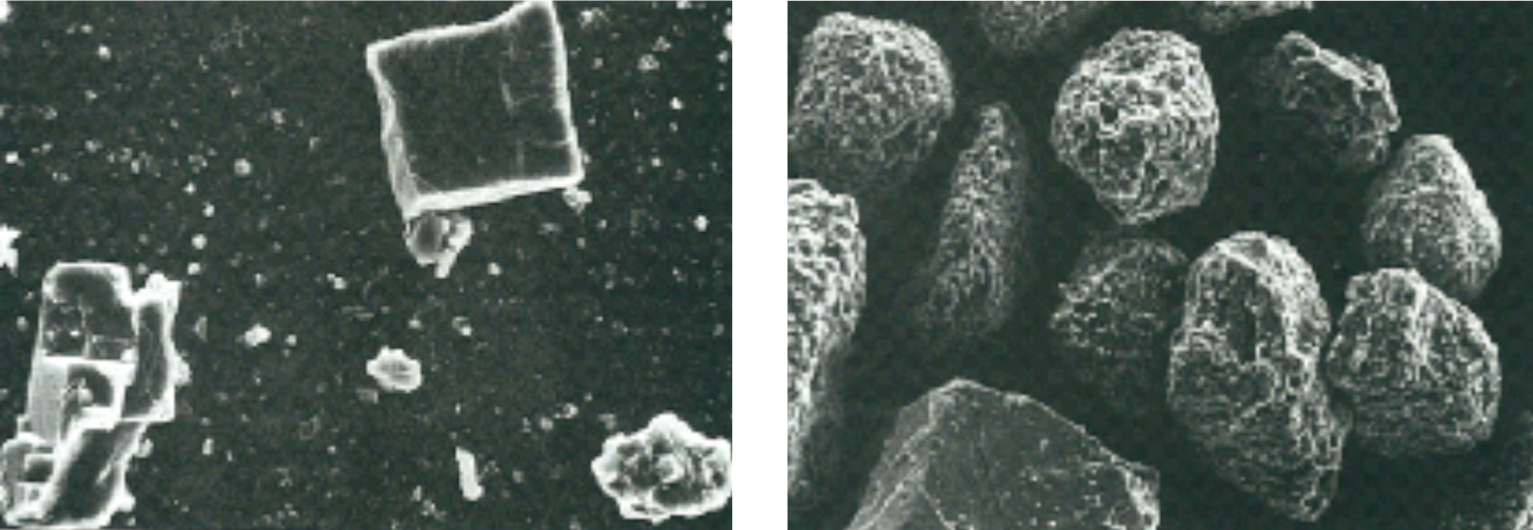3 Rates

# Log-log diagramFigure 1: Comparison of the viscosity curves of three solutions and one gel for cosmetic applications: Sample 1 is a gel with a yield point because in the low-shear range the viscosity approaches infinity. Both samples 2 and 3 show a plateau value of zero-shear viscosity, and are therefore liquids when at rest.

This kind of a diagram is often used for viscosity curves of polymers with uncrosslinked molecules (Figure 1). Here, in the low-shear range, which is typically associated with shear rates below 1 s-1, each of these viscosity curves shows a constant value, which is the so-called plateau value of the zero-shear viscosity η0. This term was chosen because in this case the shear rate approaches zero and therefore the state at rest.

Sample 1: In the direction of decreasing shear rates, the viscosity of this gel increases towards infinity. This indicates a firm texture of the gel at rest, with distinct shear-thinning flow behavior towards higher shear rates. Samples 2 and 3: At rest, they are fluid and therefore self-leveling, as they both have a plateau of zero-shear viscosity. Above a shear rate of 50 s-1 or 100 s-1, respectively, they are shear-thinning. Sample 4 shows ideally viscous (or Newtonian) behavior over the entire measuring range with a viscosity of η = 22 mPas.

The occurrence of shear-thickening in suspensions should be expected under the following two conditions: at (1) high shear, e.g. caused by high shear rates, and with a (2) high amount of solid matter (better expressed as solid/volume percentage; this is the fraction of solid particles in the total dispersion volume). Particles in flowing dispersions are constantly subjected to rotational motion, therefore particle shape has a big effect. The more the shape deviates from the spherical shape, the stronger the effect (Figure 2). This is due to the different amounts of space required by rotating particles of different shape. The result can be disturbance or even blockage of uniform flow, for example in spray-coating processes.Figure 2: Effect of particle shape on flow behavior. With the same solid/volume percentage, the suspension containing the angular limestone particles (left) displays shear-thickening flow behavior at a lower shear rate than the suspension with the rounder particles (right).